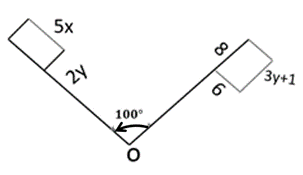Mathematics
Easy

Question

# The quadrilateral is rotated about O. What is the value of y?## 2    3    4    5Hint:

## The correct answer is: 3

### Rotation is isometry.Step 1 :Comparing corresponding parts2y = 6y = 33, is the value of y.5x = 3y + 1 (substitute y = 3)5x = 3(3) + 15x = 10x = 2

corresponding parts of quadrilaterals, remain same after rotation.

### Related Questions to study#### With Turito Foundation.#### Get an Expert Advice From Turito.